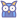## Bi-Noising Diffusion: Towards Conditional Diffusion Models with Generative Restoration Priors

• Johns Hopkins University• *denotes equal contribution### Abstract

Conditional diffusion probabilistic models can model the distribution of natural images and can generate diverse and realistic samples based on given conditions. However, oftentimes their results can be unrealistic with observable color shifts and textures. We believe that this issue results from the divergence between the probabilistic distribution learned by the model and the distribution of natural images. The delicate conditions gradually enlarge the divergence during each sampling timestep. To address this issue, we introduce a new method that brings the predicted samples to the training data manifold using a pretrained unconditional diffusion model. The unconditional model acts as a regularizer and reduces the divergence introduced by the conditional model at each sampling step. We perform comprehensive experiments to demonstrate the effectiveness of our approach on super-resolution, colorization, turbulence removal, and image-deraining tasks. The improvements obtained by our method suggest that the priors can be incorporated as a general plugin for improving conditional diffusion models.

### TL;DR

Code samples based on stable-diffusion-2

``````
noise_pred = cond_unet(latent_model_input, t, conditions=(condition)).sample
+ noise_pred, _ = noise_pred.chunk(2, dim=1)
+ pred_latents = _predict_xstart_from_eps(latent_model_input, t, noise_pred).clamp(-1, 1)
+ latent_model_input = q_sample(pred_latents, t)
+ noise_pred = uncond_unet(latent_model_input, t, conditions=(uncondition))

# classifier free guidance
- noise_pred_uncond = uncond_unet(latent_model_input, t, conditions=(uncondition)).sample
- noise_pred_uncond, noise_pred_text = noise_pred.chunk(2)
- noise_pred = noise_pred_uncond + guidance_scale * (noise_pred_text - noise_pred_uncond)
``````

### Acknowledgements

The website template was borrowed from Mip-NeRF 360.